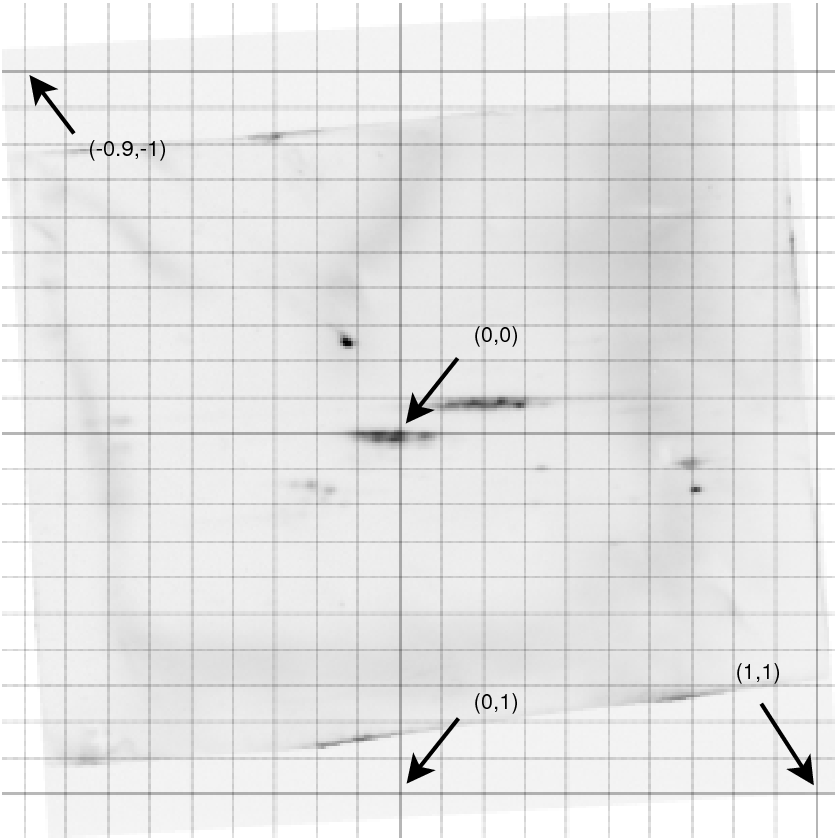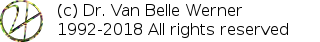Project Home

# IIS: Basic Concepts

Two considerations are important for interactive image spreadsheets. First, users want the ability to point to objects/features in the image, which requires input. Since we cannot require the user to point to the same object every time a cell needs to be calculated, interactive results are cached as long as necessary. Whenever appropriate the user can change them again. The second challenge centers around the coordinate system. For instance, the user might load images at resolutions of 300 DPI or 600 DPI. If (s)he then would select points in a 300 DPI image, then straightforward converting those positions to a 600dpi image will result in wrong positions. To resolve this problem we rely on an orthonormal coordinate system that we can alter as we go along.

## Interactive User Input

Cells can have various statuses such as 'recalculating', 'should be recalculated', 'error' and others. Two states are new compared to standard spreadsheets. These are 'require user input' and 'user input allowed'.
1. Require user input - when a formula is entered that requires user input, such as (select A0), a formula that will ask the user to select a position in the image, then the cell will be colored orange. In order to enter the user input, select the cell and go to Spreadsheet|start user input'.
2. User input allowed - when a cell that requires user input already has user input it will be colored yellow. The user can then change the input by selecting the cell and marking the cell again as 'start user input'.

## The Orthonormal Coordinate SystemBecause the spreadsheet has many operations on multiple images, it needs to know how to map a position in one image to another image. E.g; a specific calibration spot should always be at the same virtual location, regardless of the image. To achieve this, the spreadsheet associates with every image a virtual coordinate system that can be modified as necessary. This solves the problem of images that differ in translation (a calibration spot located at different positions), scaling (a difference in time based mass seperation might lead to different zoom factors), image resolution and image aspect ratio.

The orthonormal coordinate system is drawn using horizontal and vertical lines through (-1,-1), (0,0) and (1,1). On every 0.1 step between -1 and 1 a dotted line is drawn.

 http://werner.yellowcouch.org/mailto:werner@yellowcouch.org